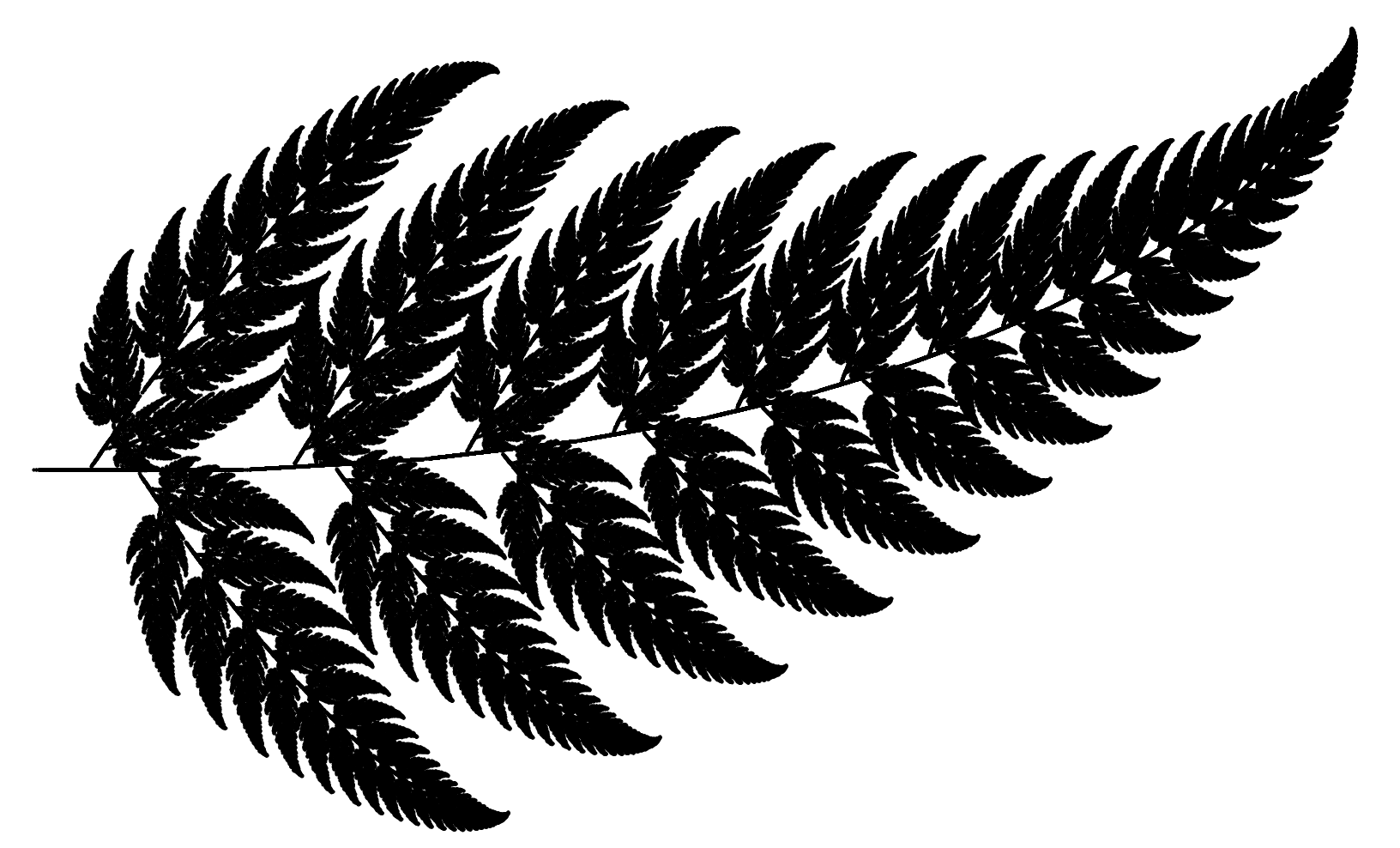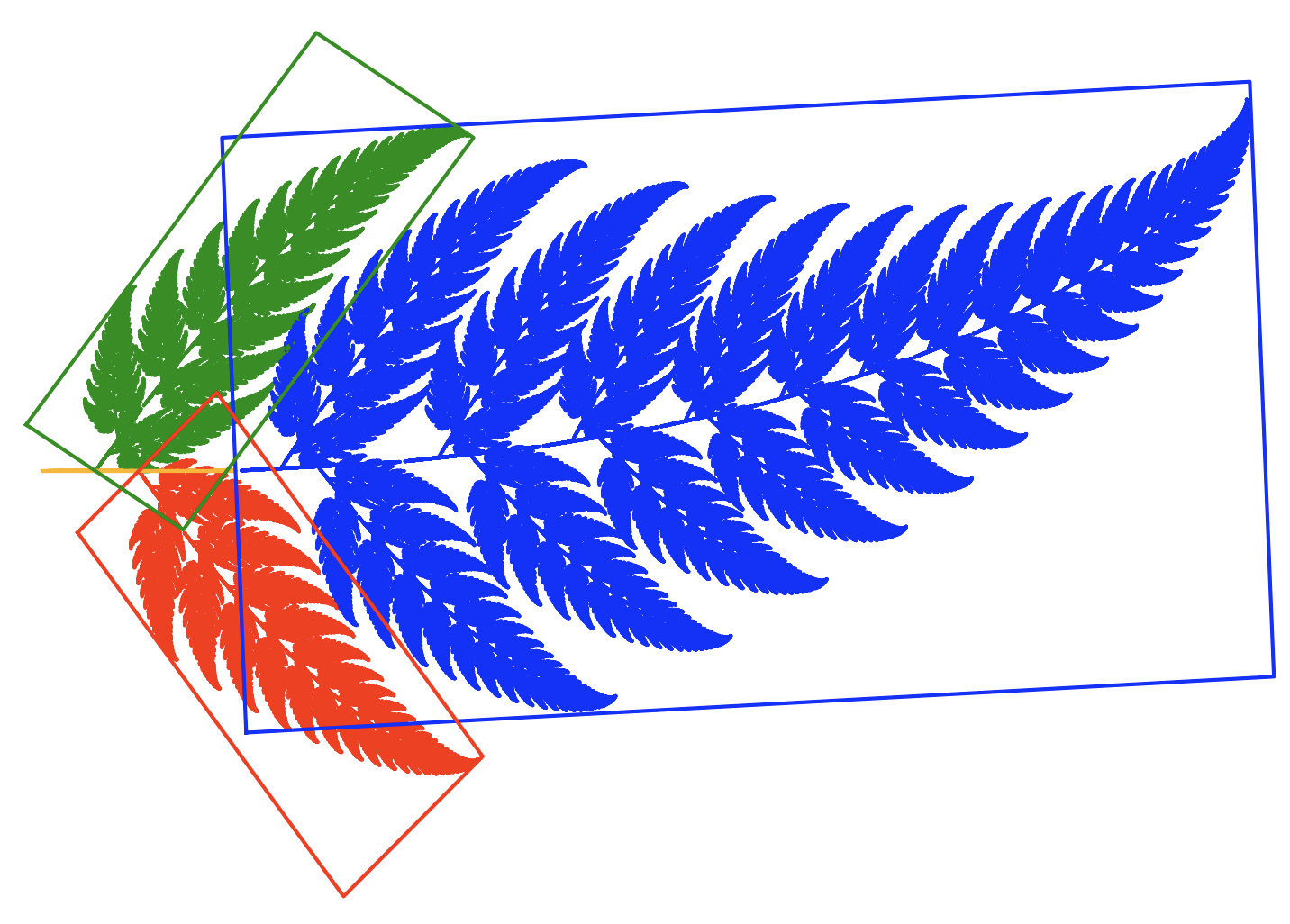## 4.1 Barnsley fernThis very popular fractal was first described only in 1993 by Michael Barnsley. The author studied the possibilities of describing various objects, mostly plants, using fractals and, in particular, iterated contraction systems. This research has led to interesting extensions of fractals, such as superfractals or parameterized V-fractals, which allow fractal figures to be mixed and mutated. A description and examples are available in the paper V-variable fractals and superfractals.

The basic version of the fern is based on the four transformations shown below.Transformations for Barnsley ferns. Color indicates the images resulting from successive transformations.

Graph obtained with the chaos game algorithm with probabilities $$\pi = [0.01; 0.79; 0.1; 0.1]$$.

$f_1(x, y) = \begin{bmatrix} 0 & 0 & 0 \\ 0 & 0.16 & 0 \end{bmatrix} [x \ y \ 1]^T$ $f_2(x, y) = \begin{bmatrix} 0.85 & 0.04 & 0 \\ -0.04 & 0.85 & 1.6 \end{bmatrix} [x \ y \ 1]^T$

$f_3(x, y) = \begin{bmatrix} 0.2 & -0.26 & 0 \\ 0.23 & 0.22 & 0.8 \end{bmatrix} [x \ y \ 1]^T$ $f_4(x, y) = \begin{bmatrix} -0.15 & 0.28 & 0 \\ 0.26 & 0.24 & 0.44 \end{bmatrix} [x \ y \ 1]^T$Experiments with ferns. Variations on the fern theme with slightly modified transformations.